Markov Model

Markov ModelState, STransition Probability, A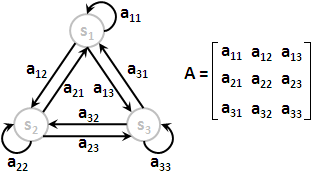Initial Probability, ΠState Sequence, q₁ q₂ ...... qT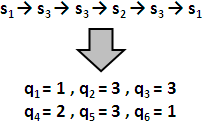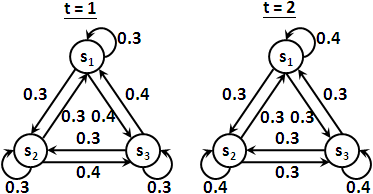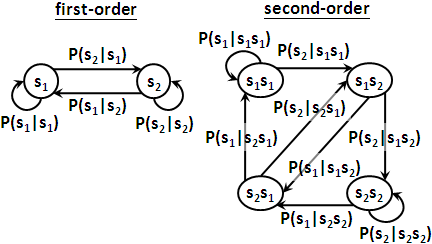Hidden Markov Model

Observation, VObservation Probability, BObservation Sequence, o₁ o₂ ...... oT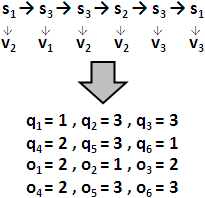Hidden Markov Model「隱藏」二字便是指行蹤被隱藏了，狀態序列被隱藏了。

1. Evaluation Problem: Forward-backward Algorithm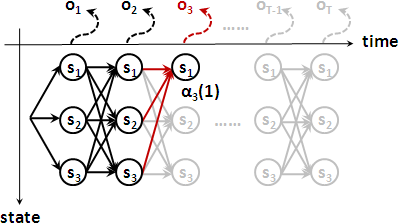```forward:
α₁(j) = πⱼ × bⱼ(o₁)
αt+1(j) = ∑i=1⋯N[ αₜ(i) × aᵢⱼ ] × bⱼ(ot+1)

backward:
βT(i) = 1
βₜ(i) = ∑j=1⋯N[ aᵢⱼ × bⱼ(ot+1) × βt+1(j) ]
```

2. Decoding Problem: Viterbi Algorithm```forward:
δ₁(j) = πⱼ × bⱼ(o₁)
δt+1(j) = maxi=1⋯N[ δₜ(i) × aᵢⱼ ] × bⱼ(ot+1)

path tracing:
ψₜ(j) = arg maxi=1⋯N[ δt-1(i) × aᵢⱼ ] × bⱼ(oₜ)
= arg maxi=1⋯N[ δt-1(i) × aᵢⱼ ]
```

3. Training Problem: EM Algorithm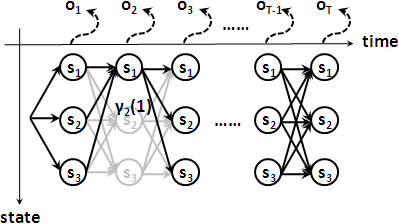```分子是穿越狀態sᵢ的所有路徑的機率的總和：

αₜ(i) × βₜ(i)
γₜ(i) = ————————————————————————— = ∑j=1⋯N ξₜ(i,j)
∑i=1⋯N [ αₜ(i) × βₜ(i) ]

αₜ(i) × aᵢⱼ × bⱼ(ot+1) × βt+1(j)
ξₜ(i,j) = —————————————————————————————————————————————————
∑i=1⋯N ∑j=1⋯N [ αₜ(i) × aᵢⱼ × bⱼ(ot+1) × βt+1(j) ]

πᵢ    = γ₁(i)
aᵢⱼ   = ∑t=1⋯T-1 [ ξₜ(i,j) ] ÷ ∑t=1⋯T-1 [ γₜ(i) ]
bⱼ(k) = ∑t=1⋯T, oₜ=k [ γₜ(i) ] ÷ ∑t=1⋯T [ γₜ(i) ]
```

```利用log(p)與log(q)，求出log(p+q)，一般讓底數等於10。b可以是任意數。

if p ≥ q
logb (p + q)  = log p + logb (1 + blogb q - logb p)
else
logb (p + q)  = log q + logb (1 + blogb p - logb q)
```

Training Problem針對沒出現的觀察值，更新之後機率是零。往後，Evaluation Problem與Decoding Problem針對此觀察值，算得機率均是零。也就是說，一旦變成零，就無法恢復成非零了。

Classification```   兩組調性不同的資料集
Data Set 1    Data Set 2
1. ABBCABCAABC   BBBCCBC
2. ABCABC        CCBABB
3. ABCAABC       AACCBBB
4. BBABCAB       BBABBAC
5. BCAABCCAB     CCAABAB
6. CACCABCA      BBBCCBAA
7. CABCABCA      ABBBBABA
8. CABCA         CCCCC
9. CABCA         BBAAA
```

```          1 × γ₁(i) + n × γ₁(n)(i)
πᵢ(n+1) = ——————————————————————————
1 + n

1 × ∑t=1⋯T-1 [ ξₜ(i,j) ] + n × ∑t=1⋯T-1 [ ξₜ(n)(i,j) ]
aᵢⱼ(n+1) = ——————————————————————————————————————————————————————
1 × ∑t=1⋯T [ γₜ(i) ] + n × ∑t=1⋯T [ γₜ(n)(i) ]

1 × ∑t=1⋯T, oₜ=k [ γₜ(i) ] + n × ∑t=1⋯T, oₜ=k [ γₜ(n)(i) ]
bⱼ(n+1)(k) = ———————————————————————————————————————————————————————
1 × ∑t=1⋯T [ γₜ(i) ] + n × ∑t=1⋯T [ γₜ(n)(i) ]
```

Pattern RecognitionICPC 6147

Continuous Hidden Markov Model

Gaussian Mixture Model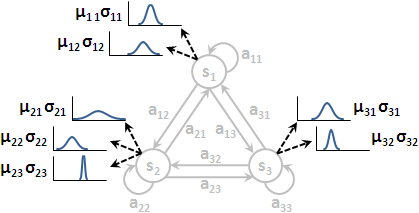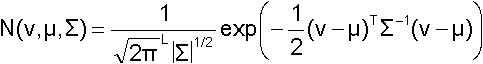```　　　路徑第一步，穿過狀態sᵢ。
πᵢ  =  γ₁(i)

分子是穿過邊aᵢⱼ。分母是穿過狀態sᵢ。
∑t=1⋯T-1 [ ξₜ(i,j) ]
aᵢⱼ = —————————————————————
∑t=1⋯T [ γₜ(i) ]

分子是穿過狀態sᵢ、使用第k個噴出管道。分母是穿過狀態sᵢ。
∑t=1⋯T [ γₜₖ(i) ]
cᵢₖ = ————————————————————————
∑t=1⋯T ∑k=1⋯M [ γₜₖ(i) ]

分子是穿過狀態sᵢ、使用第k個噴出管道、噴出數值的平均數。
∑t=1⋯T [ γₜₖ(i) ] * oₜ
μᵢₖ = ————————————————————————
∑t=1⋯T [ γₜₖ(i) ]

分子是穿過狀態sᵢ、使用第k個噴出管道、噴出數值的共變異矩陣。
∑t=1⋯T [ γₜₖ(i) ] * (oₜ - μᵢₖ)(oₜ - μᵢₖ)T
Σᵢₖ = ——————————————————————————————————————————
∑t=1⋯T [ γₜₖ(i) ]
```